# RS Aggarwal Class 9 Solutions Chapter 1 Real Numbers Ex 1E

## RS Aggarwal Class 9 Solutions Chapter 1 Real Numbers Ex 1E

These Solutions are part of RS Aggarwal Solutions Class 9. Here we have given RS Aggarwal Solutions Class 9 Chapter 1 Real Numbers Ex 1E.

Other Exercises

Rationalise the denominator of each of the followings :

Question 1.
Solution:
Here,RF of √7 is √7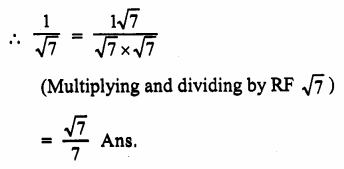Question 2.
Solution:
Here RF √3 is √3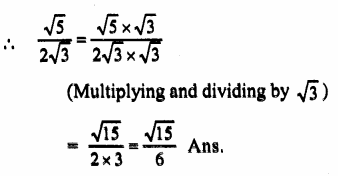Question 3.
Solution:
Here RF of $$\frac { 1 }{ \left( { 2+ }\sqrt { 3 } \right) }$$ is $$\frac { 1 }{ \left( { 2- }\sqrt { 3 } \right) }$$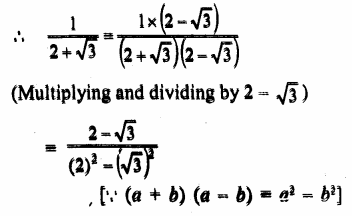Question 4.
Solution:
Here RF is √5 + 2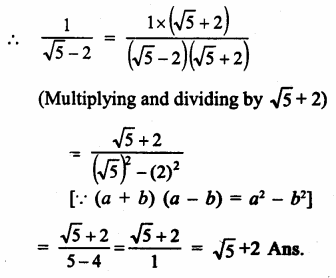Question 5.
Solution:
Here RF is 5 – 3√2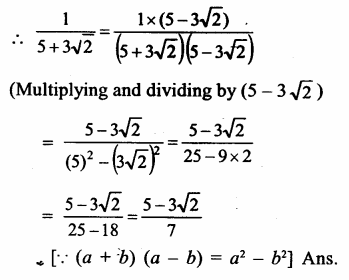Question 6.
Solution:
Here RF is √6 + √5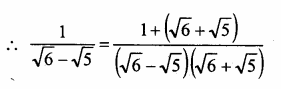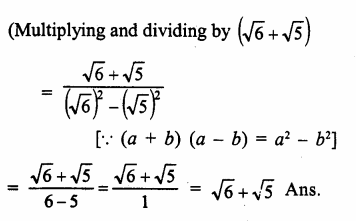Question 7.
Solution:
Here RF = √7 – √3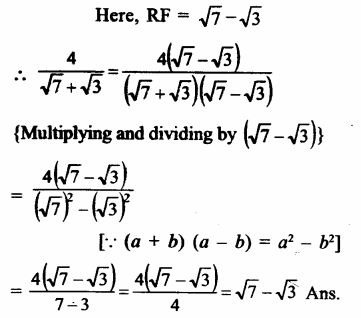Question 8.
Solution:
Here RF = √3 – 1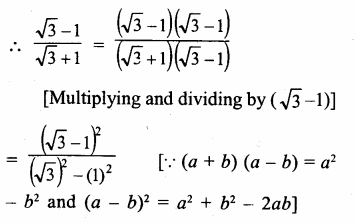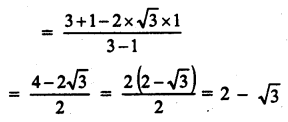Question 9.
Solution:
Here RF = (3-2√2)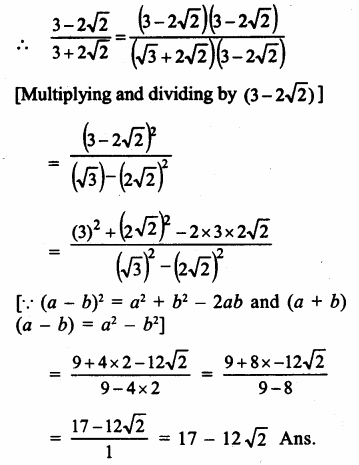Find the values of a and b in each of the following :

Question 10.
Solution:
$$\frac { \sqrt { 3 } +1 }{ \sqrt { 3 } -1 }$$, RF = √3+1
(Multiplying and dividing by √3+1)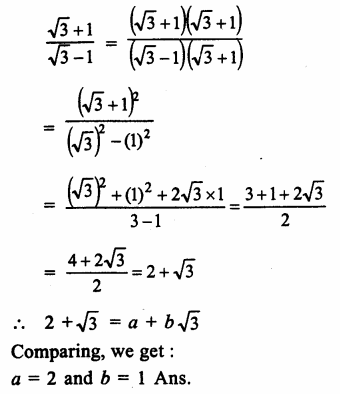Question 11.
Solution:
$$\frac { 3+\sqrt { 2 } }{ 3-\sqrt { 2 } }$$, RF is 3+√2
(Multiplying and dividing by 3+√2)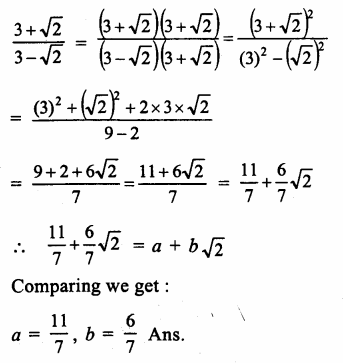Question 12.
Solution:
In $$\frac { 5-\sqrt { 6 } }{ 5+\sqrt { 6 } }$$, RF is (5-√6)
(Multiplying and dividing by 5-√6)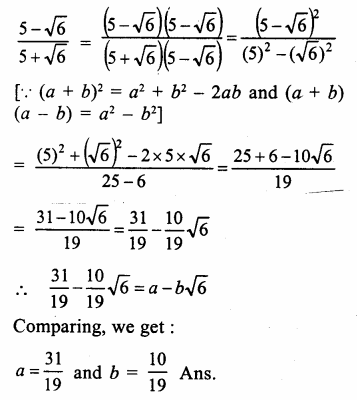Question 13.
Solution:
In $$\frac { 5+2\sqrt { 3 } }{ 7+4\sqrt { 3 } }$$, RF is 7-4√3
(Multiplying and dividing by 7-4√3)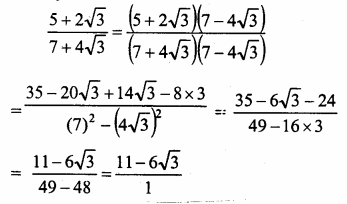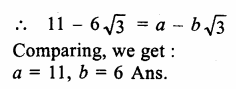Question 14.
Solution: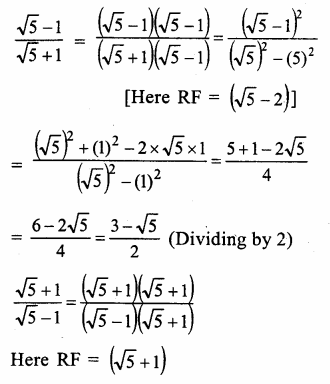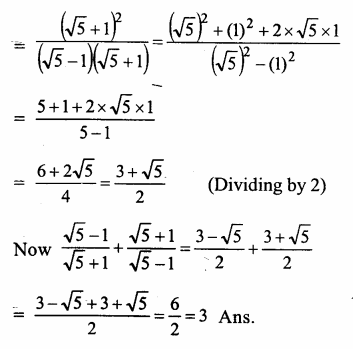Question 15.
Solution: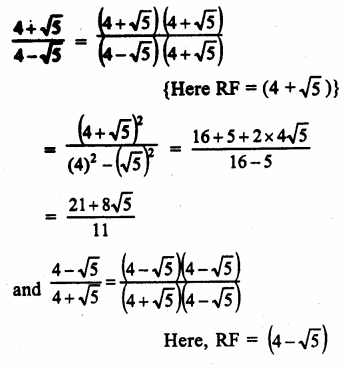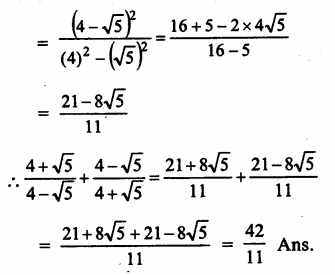Question 16.
Solution:
x = (4-√15)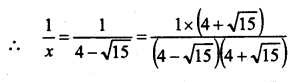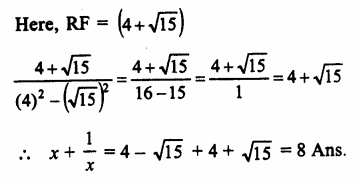Question 17.
Solution:
x = 2+√3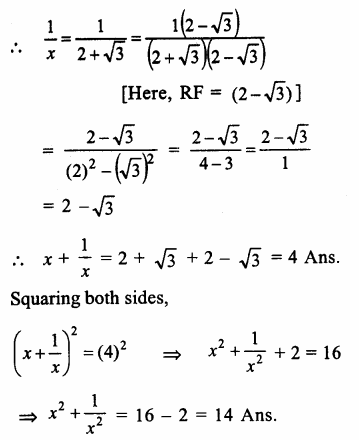Question 18.
Solution: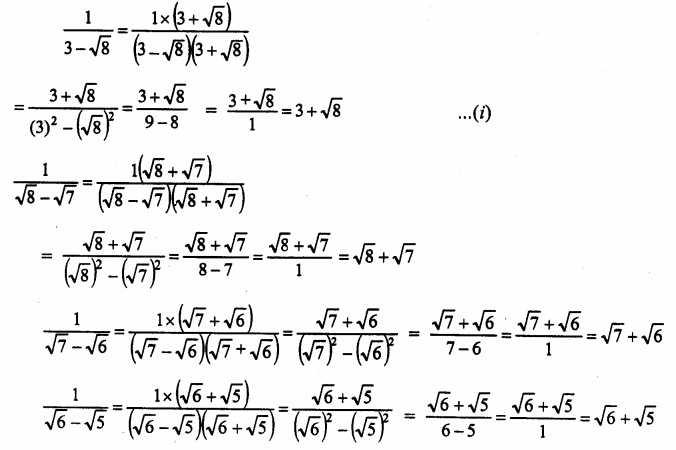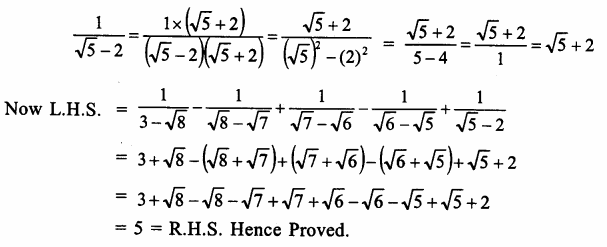Hope given RS Aggarwal Solutions Class 9 Chapter 1 Real Numbers Ex 1E are helpful to complete your math homework.

If you have any doubts, please comment below. Learn Insta try to provide online math tutoring for you.